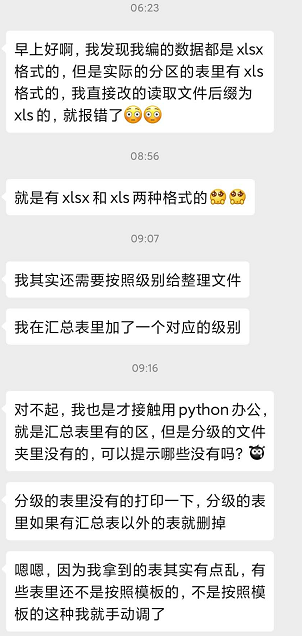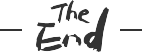Pandas案例精进 | 自动分割汇总表写入到子表Aidan075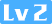160 0 0## Pandas案例需求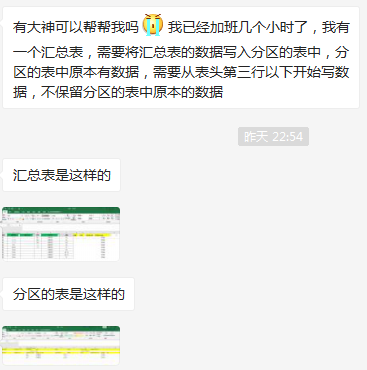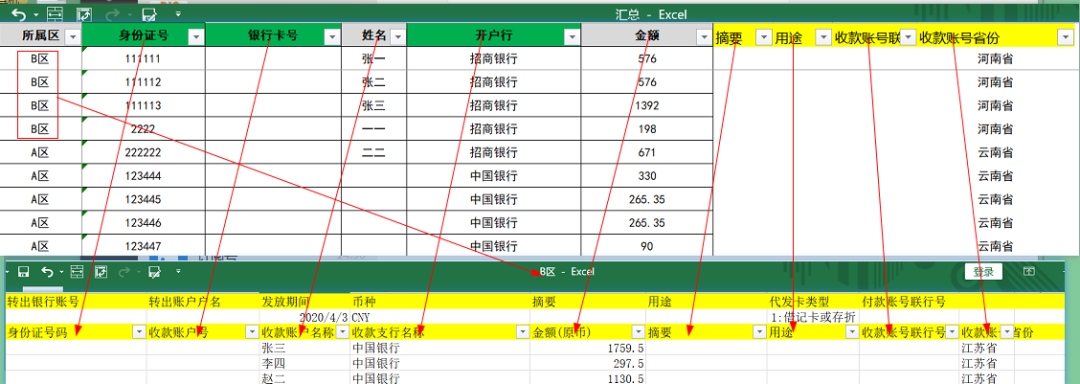B区.xlsx在自动填入后，结果如下：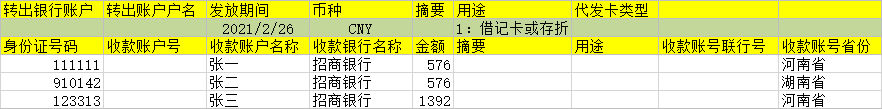## 汇总表的数据情况

import pandas as pd
data = pd.read_excel("汇总.xlsx", sheet_name='明细')
data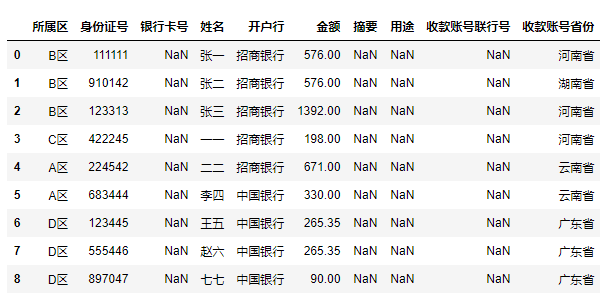## 筛选出准备写入B区的数据

df = data[data["所属区"] == "B区"]
df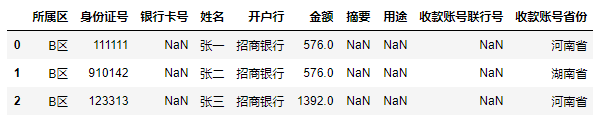df = df.iloc[:, 1:]
df.values.tolist()


[[111111, nan, '张一', '招商银行', 576.0, nan, nan, nan, '河南省'],
[111112, nan, '张二', '招商银行', 576.0, nan, nan, nan, '湖南省'],
[111113, nan, '张三', '招商银行', 1392.0, nan, nan, nan, '河南省']]


## 覆盖写入到对应的分区文件

workbook = load_workbook(filename="B区.xlsx")
sheet = workbook.active


sheet.delete_rows(idx=4, amount=1000)


for row in df.values.tolist():
sheet.append(row)
workbook.save(filename="B区.xlsx")
workbook.close()## 遍历分区字段的简单办法

for area, df in data.groupby('所属区'):
print(area)
display(df)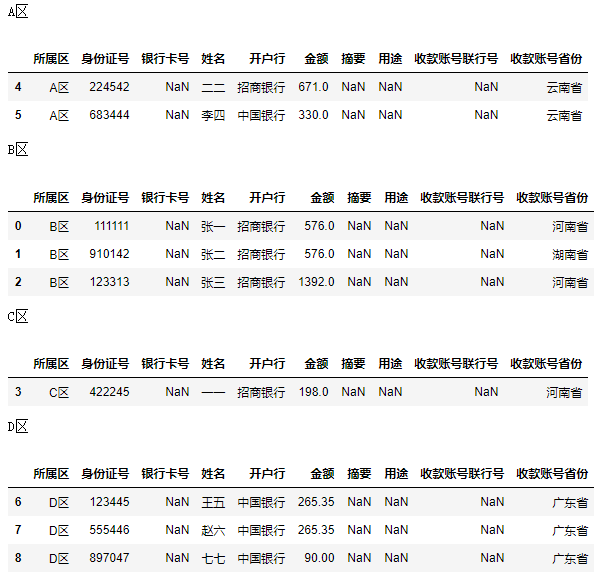## 完整代码

data = pd.read_excel("汇总.xlsx", sheet_name='明细')

for area, df in data.groupby('所属区'):
print(area)
if os.path.exists(f"{area}.xlsx"):
workbook = load_workbook(filename=f"{area}.xlsx")
else:
print(f"{area}.xlsx不存在")
continue
sheet = workbook.active
df = df.iloc[:, 1:]
# 先删除第4行之后的旧数据，预计1000行完全够用
sheet.delete_rows(idx=4, amount=1000)
# 然后在进行添加数据
for row in df.values.tolist():
sheet.append(row)
print(row)
print(f"保存到{area}.xlsx文件中")
workbook.save(filename=f"{area}.xlsx")
workbook.close()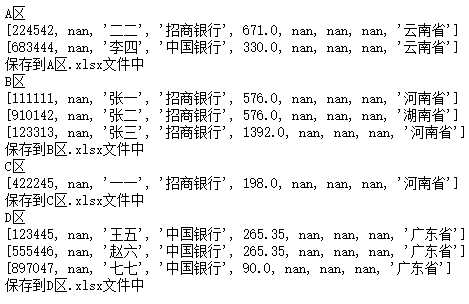### 本文代码和案例数据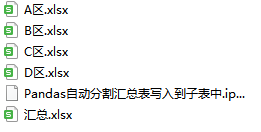## 后续